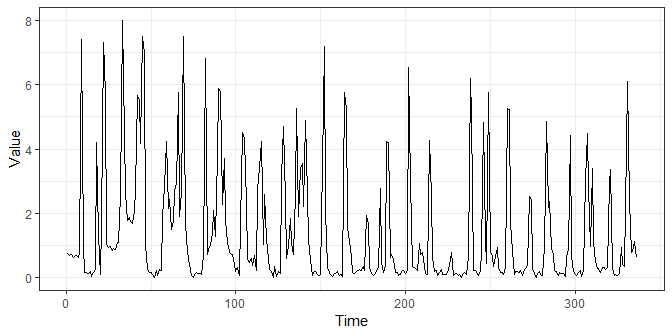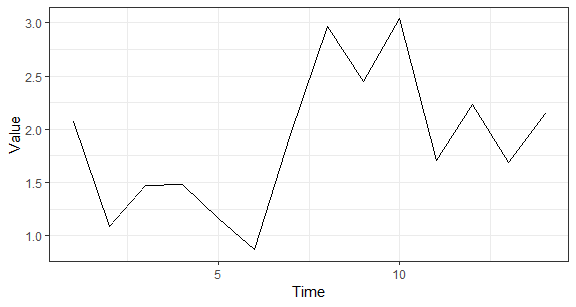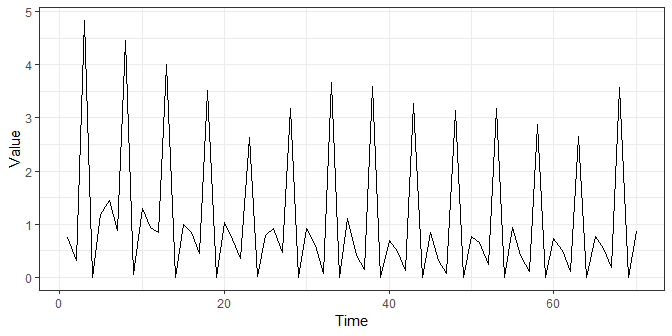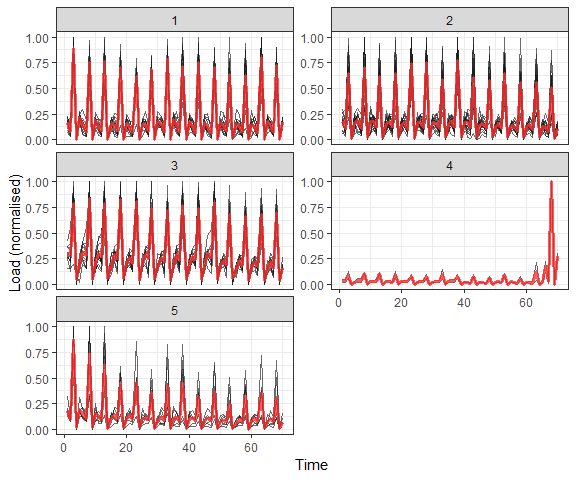TSrepr: Simple extensible framework

2019-05-31

In this vignette (tutorial), I want to demonstrate you, how the TSrepr package is simply extensible. Its methods (functions) can be extended (or combined) for arbitrary feature extraction method from a time series or by a new time series representation method. This useful feature supports several implemented functions in TSrepr package. They can be split into two groups according to a number of features extracted:

• extract only one value (feature) from a time series (or time series subsequence)
• extract multiple values (features) from a time series (or time series subsequence)

The first of the mentioned scenarios supports methods (functions): PAA (repr_paa), Mean Seasonal Profile (repr_seas_profile) and FeaTrend (repr_featrend). The second scenario supports functions: repr_windowing and repr_matrix.

The PAA representation method aggregates subsequence of a time series by one value - in original by an average value. However, it can be also used for extracting other useful features. For example, it can be median, sum or minimum and maximum. For instance, we want to aggregate (sum) pairs of values in a time series. Let’s show it on real data:

library(TSrepr)
library(ggplot2)
## Warning: package 'ggplot2' was built under R version 3.5.3
length(data_ts)
##  672
data_ts_sums <- repr_paa(data_ts, q = 2, func = sum)
length(data_ts_sums)
##  336
ggplot(data.frame(Time = 1:length(data_ts_sums), Value = data_ts_sums), aes(Time, Value)) +
geom_line() +
theme_bw()We can also extract some advanced useful features from a time series like skewness or kurtosis (implemented in package moments). Let’s extract skewness from every day of the time series (frequency is 48).

library(moments)

data_ts_skew <- repr_paa(data_ts, q = 48, func = skewness)

ggplot(data.frame(Time = 1:length(data_ts_skew), Value = data_ts_skew), aes(Time, Value)) +
geom_line() +
theme_bw()The second scenario is extracting multiple values (features) from a subsequence of time series. Here, we can use windowing method that is implemented by repr_windowing function. There is just one simple restriction for a custom representation method function and that it must return a vector. Let’s create function (repr_fea_extract) that will extract some basic features from a time series.

repr_fea_extract <- function(x) {
return(c(mean(x), median(x), max(x), min(x), sd(x)))
}

And use it with windowing function on our data.

data_fea <- repr_windowing(data_ts, win_size = 48, func = repr_fea_extract)

ggplot(data.frame(Time = 1:length(data_fea), Value = data_fea), aes(Time, Value)) +
geom_line() +
theme_bw()I will show you now, how to apply it on whole dataset (by function repr_matrix), cluster final representations and then how to interpret results. Before applying clustering on electricity consumption data, normalisation is needed. We can use classical z-score (norm_z) or min-max (norm_min_max) normalisation methods for every consumers time series. However, there is a possibility to use directly, in function repr_matrix, arbitrary defined normalisation function. For instance, let’s use a simple self-defined max normalisation.

norm_max <- function(x) {
return(x/max(x))
}
data_mat <- repr_matrix(elec_load, func = repr_fea_extract,
windowing = T, win_size = 48, normalise = T,
func_norm = norm_max)

set.seed(123)
clus_res <- kmeans(data_mat, centers = 5, nstart = 10)

Let’s plot the final clusters with corresponding centroids (red line).

library(data.table)
# prepare data for plotting
data_plot <- data.table(melt(data.table(class = as.factor(clus_res$cluster), data_mat))) data_plot[, Time := rep(1:ncol(data_mat), each = nrow(data_mat))] data_plot[, ID := rep(1:nrow(data_mat), ncol(data_mat))] # prepare centroids centers <- data.table(melt(clus_res$centers))
setnames(centers, c("Var1", "Var2"), c("class", "Time"))
centers[, ID := class]

# plot the results
ggplot(data_plot, aes(Time, value, group = ID)) +
facet_wrap(~class, ncol = 2, scales = "free_y") +
geom_line(color = "grey10", alpha = 0.65) +
geom_line(data = centers, aes(Time, value), color = "firebrick1", alpha = 0.80, size = 1.2) +
labs(x = "Time", y = "Load (normalised)") +
theme_bw()Let’s see also frequency table of occurrence in clusters.

table(clus_res\$cluster)
##
##  1  2  3  4  5
## 12 17 12  2  7

There are three dominant clusters (n. 1, 2 and 3). Time series in clusters n. 4 and 5 are irregular against other time series, so they were assigned to own clusters.

In this vignette, I showed you how simple it is to use arbitrary functions for feature extraction from time series in order to create your own time series representations alongside implemented methods in the package TSrepr.